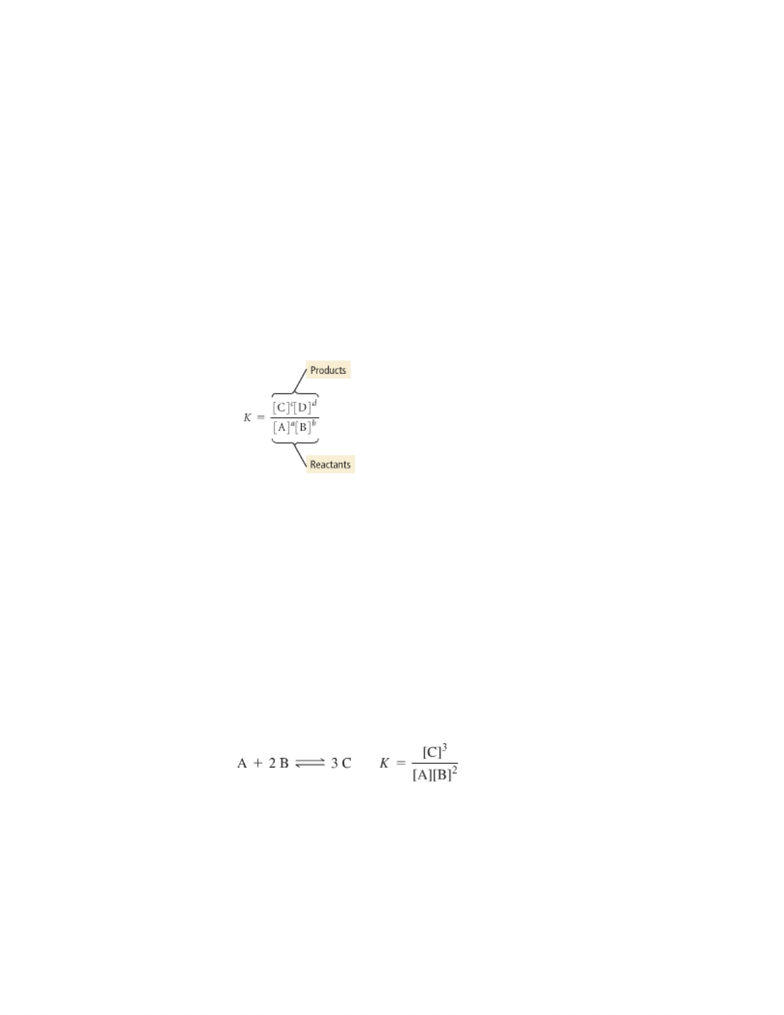# Chapter 14--Equilibrium: Key Terms & Concepts

45 views3 pagesChapter 14: Chemical Equilibrium
Dynamic Equilibrium:
The condition in which the rate of the forward reaction equals the rate of the
reverse reaction
As concentration of product increases, and concentrations of reactants
decrease, rate of forward reaction slows down and rate of reverse reaction
speeds up
Law of Mass Action: ex: aA + bB < -- > cC + dD
K < 1, reverse reaction is favored
K = 1, neither direction is favored
K > 1, forward reaction is favored
If you reverse the equation, invert the equilibrium.
k(reverse) = 1/k(forward)
if you multiply the coefficients in the equation by a factor, raise the
equilibrium constant to the same factor :
if you have two equilibrium equations, multiple k1 and k2 to obtain the
overall k
k3 = k1 x k2
www.notesolution.com
Unlock document

This preview shows page 1 of the document.
Unlock all 3 pages and 3 million more documents.

## Document Summary

 the condition in which the rate of the forward reaction equals the rate of the reverse reaction.  as concentration of product increases, and concentrations of reactants decrease, rate of forward reaction slows down and rate of reverse reaction speeds up. Law of mass action: ex: aa + bb < -- > cc + dd.  k < 1, reverse reaction is favored.  k = 1, neither direction is favored.  k > 1, forward reaction is favored. Ice tables are used to calculate initial concentrations, the change in concentration, and the equilibrium concentration.  reactions quotient (qc & qp) is the ratio of the concentrations of the products raised to their stoichiometric coefficients divided by the concentration of the reactants raised to the their stoichiometric coefficients.  the value of q relative to k is a measure of the progress of the reaction toward equilibrium. At equilibrium, the reaction quotient is equal to the equilibrium constant.

# Get access

\$10 USD/m
Billed \$120 USD annually
Homework Help
Class Notes
Textbook Notes